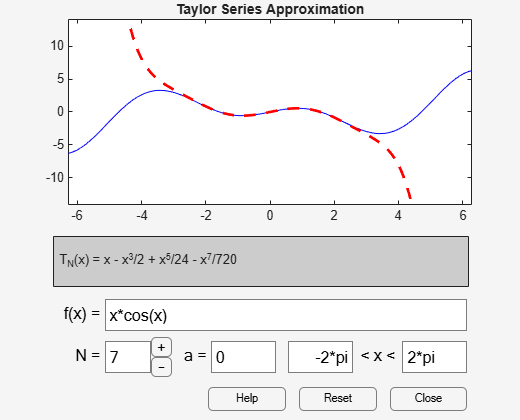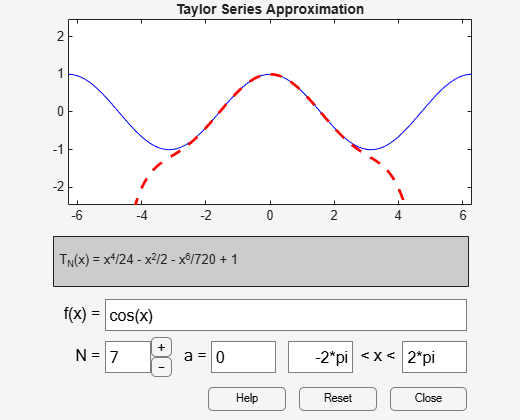# taylortool

Taylor series calculator

## Syntax

``taylortool``
``taylortool(f)``

## Description

example

````taylortool` initiates a GUI that computes the Taylor series expansion. The GUI that graphs a function against the Nth partial sum of its Taylor series about a base point `x = a`. The default function, value of N, base point, and interval of computation for `taylortool` are ```f = x*cos(x)```, `N = 7`, `a = 0`, and `[-2*pi,2*pi]`, respectively.```

example

````taylortool(f)` initiates the GUI that computes the Taylor series expansion of the given expression `f`.```

## Examples

collapse all

Open a GUI that computes the Taylor series expansion. By default, the GUI shows the Taylor series expansion of the function `f(x) = x*cos(x)`. The blue solid line shows the function `f(x)` and the red dashed line shows its Taylor approximation.

`taylortool`Next, find the Taylor series expansion of the function `cos(x)`.

```syms x taylortool(cos(x))```## Input Arguments

collapse all

Input to approximate, specified as a symbolic expression or function.

## Version History

Introduced before R2006a